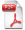DetailsVibrations of Linear Piezostructures

Wiley-ASME Press Series 1. Aufl.
 81,99 € Verlag: Wiley Format: PDF Veröffentl.: 07.05.2021 ISBN/EAN: 9781119393504 Sprache: englisch Anzahl Seiten: 256

DRM-geschütztes eBook, Sie benötigen z.B. Adobe Digital Editions und eine Adobe ID zum Lesen.

Beschreibungen

Titelbeschreibung

<p><b>A thorough guide to the fundamental development of linear piezoelectricity for vibrations </b></p> <p><i>Vibrations of Linear Piezostructures</i> is an introductory text that offers a concise examination of the general theory of vibrations of linear piezostructures. This important book brings together in one comprehensive volume the most current information on the theory for modeling and analysis of piezostructures.  The authors explore the fundamental principles of piezostructures, review the relevant mathematics, continuum mechanics and elasticity, and continuum electrodynamics as they are applied to electromechanical piezostructures, and include the work that pertains to linear constitutive laws of piezoelectricity.  </p> <p>The book addresses modeling of linear piezostructures via Newton’s approach and Variational Methods.  In addition, the authors explore the weak and strong forms of the equations of motion, Galerkin approximation methods for the weak form, Fourier or modal methods, and finite element methods. This important book: </p> <ul> <li>Covers the fundamental developments to vibrational theory for linear piezostructures  </li> <li>Provides an introduction to continuum mechanics, elasticity, electrodynamics, variational calculus, and applied mathematics </li> <li>Offers in-depth coverage of Newton’s formulation of the equations of motion of vibrations of piezo-structures </li> <li>Discusses the variational methods for generation of equations of motion of piezo-structures </li> </ul> <p>Written for students, professionals, and researchers in the field, <i>Vibrations of Linear Piezostructures</i> is an up-to-date volume to the fundamental development of linear piezoelectricity for vibrations from initial development to fully modeled systems using various methods. </p>

Inhaltsverzeichnis

<p>1.1 The Piezoelectric Effect 13</p> <p>1.1.1 Ferroelectric Piezoelectrics 14</p> <p>1.1.2 One Dimensional Direct and Converse Piezoelectric Effect 17</p> <p>1.2 Applications 19</p> <p>1.2.1 Energy Applications 19</p> <p>1.2.2 Sensors 20</p> <p>1.2.3 Actuators or Motors 20</p> <p>1.3 Outline of the Book 22</p> <p>2 Mathematical Background 27</p> <p>2.1 Vectors, Bases, and Frames 27</p> <p>2.2 Tensors 29</p> <p>2.3 Symmetry, Crystals, and Tensor Invariance 33</p> <p>2.3.1 Geometry of Crystals 33</p> <p>2.3.2 Symmetry of Tensors 41</p> <p>2.4 Problems 43</p> <p>3 Review of Continuum Mechanics 45</p> <p>3.1 Stress 45</p> <p>3.1.1 The Stress Tensor 46</p> <p>3.1.2 Cauchy’s Formula 47</p> <p>3.1.3 The Equations of Equilibrium 48</p> <p>3.2 Displacement and Strain 49</p> <p>3.3 Strain Energy 55</p> <p>3.4 Constitutive Laws for Linear Elastic Materials 56</p> <p>3.4.1 Triclinic Materials 59</p> <p>3.4.2 Monoclinic Materials 60</p> <p>3.4.3 Orthotropic Materials 60</p> <p>3.4.4 Transversely Isotropic Materials 60</p> <p>3.5 The Initial–Boundary Value Problem of Linear Elasticity 61</p> <p>3.6 Problems 63</p> <p>4 Review of Continuum Electrodynamics 65</p> <p>4.1 Charge and Current 65</p> <p>4.2 The Electric and Magnetic Fields 66</p> <p>4.2.1 The Definition of the Static Electric Field 66</p> <p>4.2.2 The Definition of the Static Magnetic Field 67</p> <p>4.3 Maxwell’s Equations 69</p> <p>4.3.1 Polarization and Electric Displacement 69</p> <p>4.3.2 Magnetization and Magnetic Field Intensity 73</p> <p>4.3.3 Maxwell’s Equations in Gaussian Units 75</p> <p>4.3.4 Scalar and Vector Potentials 76</p> <p>4.4 Problems 77</p> <p>5 Linear Piezoelectricity 81</p> <p>5.1 Constitutive Laws of Linear Piezoelectricity 81</p> <p>5.2 The Initial–Value Boundary Problem of Linear Piezoelectricity 84</p> <p>5.2.1 Piezoelectricity and Maxwell’s Equations 84</p> <p>5.2.2 The Initial–Boundary Value Problem 85</p> <p>5.3 Thermodynamics of Constitutive Laws 87</p> <p>5.4 Symmetry of Constitutive Laws for Linear Piezoelectricity 91</p> <p>5.4.1 Monoclinic C2 Crystals 92</p> <p>5.4.2 Monoclinic Cs Crystals 93</p> <p>5.4.3 Trigonal D3 Crystals 94</p> <p>5.4.4 Hexagonal C6v Crystals 94</p> <p>5.5 Problems 95</p> <p>6 Newton’s Method for Piezoelectric Systems 97</p> <p>6.1 An Axial Actuator Model 97</p> <p>6.2 An Axial, Linear Potential, Actuator Model 102</p> <p>6.3 A Linear Potential, Beam Actuator 104</p> <p>6.4 Composite Plate Bending 108</p> <p>6.5 Problems 116</p> <p>7 Variational Methods 119</p> <p>7.1 A Review of Variational Calculus 119</p> <p>7.2 Hamilton’s Principle 122</p> <p>7.2.1 Uniaxial Rod 123</p> <p>7.2.2 Bernoulli-Euler Beam 125</p> <p>7.3 Hamilton’s Principle for Piezoelectricity 126</p> <p>7.3.1 Uniaxial Rod 130</p> <p>7.3.2 Bernoulli-Euler Beam 132</p> <p>7.4 Bernoulli-Euler Beam with a Shunt Circuit 133</p> <p>7.5 Relationship to other Variational Principles 140</p> <p>7.6 Lagrangian Densities 143</p> <p>7.7 Problems 151</p> <p>8 Approximations 153</p> <p>8.1 Classical, Strong, and Weak Formulations 153</p> <p>8.2 Modeling Damping and Dissipation 161</p> <p>8.3 Galerkin Approximations 163</p> <p>8.3.1 Modal or Eigenfunction Approximations 167</p> <p>8.3.2 Finite Element Approximations 179</p> <p>8.4 Problems 200</p> <p>Supplementary Material 201</p> <p>S.1 A Review of Vibrations 201</p> <p>S.1.1 SDOF Systems 201</p> <p>S.1.2 Distributed Parameter Systems 205</p> <p>S.1.3 MDOF Equations of Motion 219</p> <p>S.2 Tensor Analysis 222</p> <p>S.3 Distributional and Weak Derivatives 224</p>

Autorenportrait

<p><b>Andrew J. Kurdila </b>earned his PhD in Engineering Science and Mechanics in 1989 from the Georgia Institute of Technology, USA. Currently, he<b> </b>is the W. Martin Johnson Professor of Mechanical Engineering at Virginia Tech. His areas of specialty include robotics and autonomous and dynamical systems. </p> <p><b>Pablo A. Tarazaga</b> earned his PhD in 2009 from Virginia Tech, USA and is currently the Director of the Vibrations, Adaptive Structures and Testing Laboratory and the Director of the Virginia Tech Smart Infrastructure Laboratory at Virginia Tech. His research interests include structural mechanics and dynamics and control.  </p>

Diese Produkte könnten Sie auch interessieren:Turbulent Drag Reduction by Surfactant Additives
von: Feng-Chen Li, Bo Yu, Jin-Jia Wei, Yasuo Kawaguchi142,99 €Turbulent Drag Reduction by Surfactant Additives
von: Feng-Chen Li, Bo Yu, Jin-Jia Wei, Yasuo Kawaguchi142,99 €Wear
von: Gwidon W. Stachowiak144,99 €